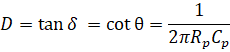## What is Loss Tangent?

PCB BasicsEditorial Team - PCB Directory

Jul 13, 2019

In PCBs, Loss tangent (tan (δ)) is the measure of signal loss due to the inherent dissipation of electromagnetic energy in the substrate of the printed circuit board. It is a dimensionless quantity and generally referred to: Loss Factor, Tan δ, Dissipation factor and Loss angle.

All PCB materials have both conduction losses and dielectric losses. The conduction losses are resistive and are caused by the conductive copper layer used in the board. The dielectric losses on the other hand are associated with the substrate (insulating material) used in a PCB.   When the electric field is applied, polarization occurs and charges are displaced relative to the electric field. Dielectric losses cause a reduction in the overall electric field.The total amount of polarization that can occur in a dielectric depends upon the molecular symmetry of the insulator material and is called Dipole Moment. The effect of the dipole moment in a dielectric substrate is termed Loss Tangent.

Mathematically, the loss tangent is the phase angle between the resistive and reactive components of a system with permittivity. A low value of loss tangents results in a "fast" substrate while large value results in a "slow" substrate.The formula for Loss Tangent or the Dissipation Factor is:where δ is the loss angle, θ is the phase angle, f is the frequency, Rp is the equivalent parallel resistance, and Cp is the equivalent parallel capacitance.

The signal loss by the dielectric material depends upon the material’s structure and glass-resin composition. Selection of low loss materials is the major task for manufacturers that is why they are advised to plot the attenuation equation against frequency for various material choices.

Loss tangent plays a crucial factor for very high frequencies above 1 GHz and analog signals to determine the signal attenuation.